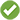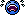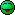# Confusion about potential energy in a tank filled with water

• Low-Q

#### Low-Q

Gold Member
I have a discussion going on with some friends. The discussion goes like this:
We have two identical tanks. One is empty. One is full of water. Both placed on the ground.
They are connected with a hose at the bottom. There is a ball valve in line with the hose.
The full tank has a given potential energy in it. The second one has zero potential energy in it because it is empty.
Now, If we open the valve, water starts to flow from one tank to the other. The potential energy increase in the empty tank, and decrease in the full tank. Finally they will level out to same amount of water. Half full each.

The flow through the hose activates a flawless turbine (for the simplicity). The turbine provides electricity to a load that heats up.
We also have an outlet hose from each tank, connected together. There is a turbine in those hoses too.
Now we open thiese outlets and let water flow from both tanks through the turbines.

The claim made from my friends is that it is possible to get 150% energy out of the tank(s). 50% potential energy from the full tank flowing into the emty one, and 100% from both tanks when opening the second valve.

Initially we discussed electric capacitors. 10uF each. One charged with 10V. Then these are connected via the load. The claim is that both capacitors finally will have 5V each. Then we empty the capacitors together with a load and they claim to achieve more energy out during discharge than we put in during charging the one capacitor.

I do not agree, but lacks a good phrase that I can use as explanation.

Hope you can help :-)

Vidar

The claim made from my friends is that it is possible to get 150% energy out of the tank(s). 50% potential energy from the full tank flowing into the emty one, and 100% from both tanks when opening the second valve.

You should know already that discussions about free energy and perpetual motion are among PF forbidden topics.

You should know already that discussions about free energy and perpetual motion are among PF forbidden topics.
I'm not discussing or claiming free energy or over unity. I need help to debunk the claim made by my friends. Free energy does not exist. I know that already :-)
So, can you, or some other help me with some equations, or other explanations or examples I can use to disprove their claim? Because I am not good at this :-)

Is it so that any topic containing the words " free energy", in spite of context, is not legal? I'm not claiming anything. Just want help to explain the issue, so I can finally disprove the claim, and close the discussion.

Vidar

As a general rule, we do not allow discussions of PMM, even for the intent of debunking them. It simply takes too much monitoring to keep such discussions from going off the deep end.

Personally, I do not object to this particular thread, as you are not trying to promote a PMM, and because yo can learn some physics by understanding what is going on.

Lets start by simplifying the system, and remove the turbine in-between the tanks. Do you really still have the same amount of potential energy at the end of the transfer than you did at the beginning? (Hint: what is the equation for gravitational potential energy?)

If the weight of all the liquid to start with in the first tank is 2w, the datum of potential energy is at the base of the tank, and the initial height of liquid in the first tank is H, what is the initial potential energy? If w is transferred to the 2nd tank so that the height in each tank is now H/2, what is the change in potential energy of the liquid? If all the liquid is now drained from the two tanks, what is the change in potential energy of the liquid?

Thanks!

Ofcourse. The ground will not longer be the reference level. Because as the 2.nd tank fills up, so does the reference level.
Since the choise is to fill another tank, the 1.st tank really have 1/2 of the potential energy.

Say the tanks measure 1x1x1m. Center of mass is 0.5 meter above ground. If the potential energy is expressed in units, say the 1.st tank isolated have potential energy of 500 units.
However, we want to fill up the other tank. That will leave a potential energy of 250 units because the reference level gradually rise.
When both tanks are half full, the center of mass is 0.25 meter above ground but contains half the weight each. That would be 125 units each. 250 units combined. Energy is preserved.

Does this make sense? Can I slam this argument into the table and say "Hey! Look, here is my argument!"?

:-)

Vidar

If the weight of all the liquid to start with in the first tank is 2w, the datum of potential energy is at the base of the tank, and the initial height of liquid in the first tank is H, what is the initial potential energy? If w is transferred to the 2nd tank so that the height in each tank is now H/2, what is the change in potential energy of the liquid? If all the liquid is now drained from the two tanks, what is the change in potential energy of the liquid?

Thanks!

Ofcourse. The ground will not longer be the reference level. Because as the 2.nd tank fills up, so does the reference level.
Since the choise is to fill another tank, the 1.st tank really have 1/2 of the potential energy.

Say the tanks measure 1x1x1m. Center of mass is 0.5 meter above ground. If the potential energy is expressed in units, say the 1.st tank isolated have potential energy of 500 units.
However, we want to fill up the other tank. That will leave a potential energy of 250 units because the reference level gradually rise.
When both tanks are half full, the center of mass is 0.25 meter above ground but contains half the weight each. That would be 125 units each. 250 units combined. Energy is preserved.

Does this make sense? Can I slam this argument into the table and say "Hey! Look, here is my argument!"?

:-)

Vidar
Parts of this seem correct, but the ground reference level doesn't change. But you seem to have correctly shown that energy is concerved.

Ok. I misphrased that reference level. The ground reference does not longer apply when the reference now is on the surface of the fluid inside the other tank :-)

I got the answer I was looking for. Thanks again.

Vidar

Parts of this seem correct, but the ground reference level doesn't change. But you seem to have correctly shown that energy is concerved.

... The ground reference does not longer apply when the reference now is on the surface of the fluid inside the other tank
Why would you ever want the reference for potential energy in this problem to be anything other than the ground?

Ok. I misphrased that reference level. The ground reference does not longer apply when the reference now is on the surface of the fluid inside the other tank :-)

I got the answer I was looking for. Thanks again.

Vidar

Why would you ever want the reference for potential energy in this problem to be anything other than the ground?
Good question.
Any suggestions that might help me understand this better, is very welcome :-)

Vidar

•Chestermiller
Ok. I'll try.
Just give me a moment.

Vidar

Using water in a 1x1x1m tank. Initial potential energy is:
Ep=m×A×(h/2)×g
1000×1×0.5×9.81=4902J

When both tanks, 1 and 2, is 1/2 full:
Ep1= Ep2=
500×1×0.25×9.81=1226.25J
Eptot=Ep1+Ep2=2452.5J

When both tanks are empty the difference is:
2xEptot=4902J

Makes this sense? Seems logical to me thoug :-)

Vidar

Using water in a 1x1x1m tank. Initial potential energy is:
Ep=m×A×(h/2)×g
1000×1×0.5×9.81=4902J

When both tanks, 1 and 2, is 1/2 full:
Ep1= Ep2=
500×1×0.25×9.81=1226.25J
Eptot=Ep1+Ep2=2452.5J

When both tanks are empty the difference is:
2xEptot=4902J

Makes this sense? Seems logical to me thoug :-)

Vidar
Using the parameters I indicated in post #5,
Initial potential energy = (2w)(H/2)=wH

Change in potential energy during flow equalization between tanks ##=-w(0.75H-0.25H)=-wH/2##
Potential energy after flow equalization = wH/2

Potential energy after draining = 0
Change in potential energy during draining = -w(H/4)-w(H/4)=-wH/2

Why would you ever want the reference for potential energy in this problem to be anything other than the ground?
You can take a reference anywhere you like (we often use Infinity) but it is the change in Potential (as in Electrical Potential Difference ) that tells you the amount of work put in or Work got out.

You can take a reference anywhere you like (we often use Infinity) but it is the change in Potential (as in Electrical Potential Difference ) that tells you the amount of work put in or Work got out.
Yeah, I should have been more clear that what I meant was "you started w/ the ground as reference, stick with it"

•Chestermiller...
You can take a reference anywhere you like...
When I was in A&P school, we always used the term "datum point"... I see it's now called "datum reference" .

And, I hated weight and balance calculations... we did them all by hand... no calculators......we did them all by hand... no calculators
Log Tables were what we used.
In the first year sixth form, the school bought two hand (turn the handle) calculators and that started things off.

Why would you ever want the reference for potential energy in this problem to be anything other than the ground?
In order to expend all potential energy available from opening the valve and end with zero. But this just adds confusion and is likely what tripped-up the OP's friend.

Has anyone gone to the trouble yet, of writing down the formula of the energy needed to fill a tank to height h? I'll leave it as an 'exercise for the student' but you will find there is a factor of h2 in it, unless I am much mistaken. This is just like the Energy in a Capacitor formula, which contains V2 and which was alluded to earlier. If you connect the two tanks with a lossless pipe, there will be Kinetic Energy involved (however slowly you make the connection) and the levels will oscillate about a half height mean. If you have a 'real' pipe connection then the KE will gradually be dispersed and Half the original Potential Energy will remain.
Let's face it, this has to be the answer. We have discussed the Capacitor Problems more times than I have had hot dinners and it is a direct analogue of the tanks problem.

•gmax137
If you connect the two tanks with a lossless pipe, there will be Kinetic Energy involved (however slowly you make the connection) and the levels will oscillate about a half height mean. If you have a 'real' pipe connection then the KE will gradually be dispersed and Half the original Potential Energy will remain.
Hence my hint in post #4. The basic premise that two half-full tanks have the same GPE as a full tank is incorrect, so the rest of the discussion is moot.

•sophiecentaur
...so the rest of the discussion is moot.
No, no... this is still relevant... lol

we did them all by hand
Log Tables were what we used.
Well then, I guess we really didn't have it too bad, we could use an Eversharp... .Last edited:
•sophiecentaur
The same thing applies for two blocks, one on top of the other. The scenarios of friction or bouncing are not considered when we start off looking at these things but any transfer of energy will involve unexpected other transfers when you consider the wider implications.